# Division of Energy Between Photons and Massive Particles

One of the ideas associated with modeling the Big Bang is that the further back in time you project, the more the universe is dominated by photons. We think of today's universe as mostly matter, but the energy of the early universe was mostly photon energy with massive particles playing a very small role.

The amount of energy in radiation in today's universe can be estimated with the use of the Stefan- Boltzmann law, considering that the universe is filled with blackbody radiation at a temperature of 2.7 K. The energy density in this equilibrium radiation is given by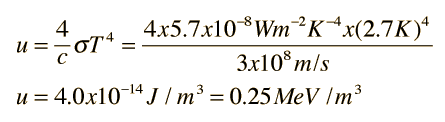There is also a background energy in neutrinos which is expected to have a temperature of about 1.9 K, and there are 7/4 as many of them as photons according to the standard model. Treating them as massless particles would give an energy density of about 0.11 MeV/m3, so the total energy density in photons and neutrinos is about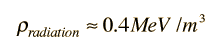More detail

One current estimate of the amount of mass in the current universe is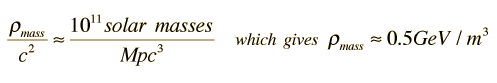,
so the current estimates place the amount of energy in massive particles as over a thousand times greater than the energy in radiation.

Note that this energy in massive particles is that of ordinary baryonic matter, but the associated density falls far short of the critical density that apparently characterizes the universe. The density is typically expressed in terms of a density parameter, Ω.

 Relate to temperature and expansion time
Index

Reference
Rohlf
Ch. 19

 HyperPhysics***** Astrophysics R Nave
Go Back

# Temperature and Expansion Time in the Standard Big Bang Model

In the big bang model of the expansion of the universe, the expansion time can be expressed in terms of the Hubble parameter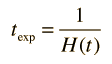,

and the Hubble parameter can be related to a model of expansion with the use of the Friedmann equation.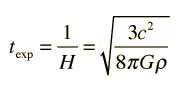.

For early stages of the expansion of the universe, it's energy density was dominated by radiation, with matter present only as a negligible contaminant. Under those conditions, the density in the Friedmann equation can be taken as that associated with the radiation field and related to the ratio of the temperature at a given time and the current temperature of the cosmic background radiation. This gives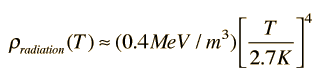.

The dependence on the fourth power of the temperature comes from the Stefan- Boltzmann law. Substituting into the Friedmann equation gives an expression of the expansion time as a function of temperature in the radiation-dominated early universe.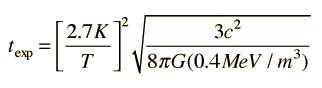.

The energy densities of radiation and matter are about equal at the temperature of the transparency point, about 3000 K. At much lower temperatures, the energy is dominated by matter. The energy density of the matter as a function of temperature is given by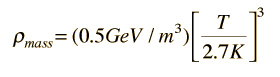.

The resulting expression for expansion time from the Friedmann equation is then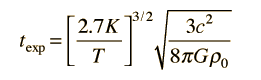.
 Calculation of expansion time Big bang time line
Index

Reference
Rohlf
Ch. 19

 HyperPhysics***** Astrophysics R Nave
Go Back

# Temperature, Expansion Time, and Energy Density in the Expanding Universe

In the radiation-dominated early universe where T>>3000K, the expansion time can be related to the temperature in the relationship:.

For temperature T = x10^ K

the corresponding expansion time is

texpansion = x10^ seconds = x10^ years.

The corresponding energy density is approximately

ρ = x10^ GeV/m3.

A characteristic photon energy is kT = x10^ MeV.

Note that this calculation includes only the photons and neutrinos, and does not apply to the times before the annihilation of most of the electrons and positrons. Another factor of 7/4 must then be introduced to include the energy contribution of the electrons and positrons.

For temperatures T<<3000K, matter dominates in energy. In the matter-dominated era, the expansion time and temperature are related by:.

Taking the matter energy density to be 0.5 GeV/m3, then the expansion time calculated from that is about 4.5 x 109 years. If the energy density of matter is taken to be the critical density of about 5.5 GeV/m3 associated with a Hubble parameter of 72 km/s/Mpc, then the expansion time at the present temperature of 2.7K is 13.6 x 109 years. The calculation of critical density comes from an expression in Weinburg. It is usually expressed in terms of a density parameter, Ω.

 A brief overview of time.
Index

References
Rohlf
Ch. 19

Weinburg

 HyperPhysics***** Astrophysics R Nave
Go Back